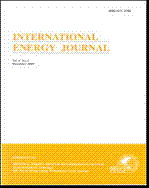## INTERNATIONAL ENERGY JOURNAL

Volume 19, No. 02, Month JUNE, Year 2019, Pages 89 - 100

#### Modeling of power characteristic curve on small scale compressed air energy storage using regression analysis

Widjonarko Widjonarko, Rudy Soenoko, Slamet Wahyudi, Eko Siswanto

### Abstract

The research on small scale compressed air energy storage (SS-CAES) becomes an interesting topic especially in optimizing the performance of the system. In this topic, the characteristic curve of the energy storage system is the key to control the system to reach optimum power to the load. In previous research, mathematical equations were used to get the characteristic curve. This paper proposes the polynomial regression based on the actual output data from the prototype to model the characteristic curve of the SS-CAES prototype. The authors have compared the use of mathematical models and polynomial regression in modeling the power curve with actual observational data and determining the level of accuracy of modeling. The results showed that by using polynomial regression, the characteristics of the SS-CAES prototype power curve could only be obtained by using the sample data from the system output with accuracy value 0.967 for R-square. Thus, an approach using this method would facilitate researchers to obtain the characteristics of the curve of the system.

### Keywords

modeling, optimization, polynomial regression, power curve, small scale compressed air energy storage (SS-CAES).

## INTERNATIONAL ENERGY JOURNAL

###### Published by : Asian Institute of TechnologyContributions welcome at : http://www.rericjournal.ait.ac.th